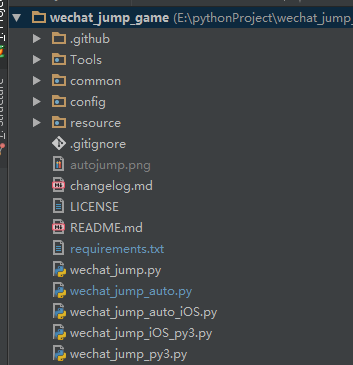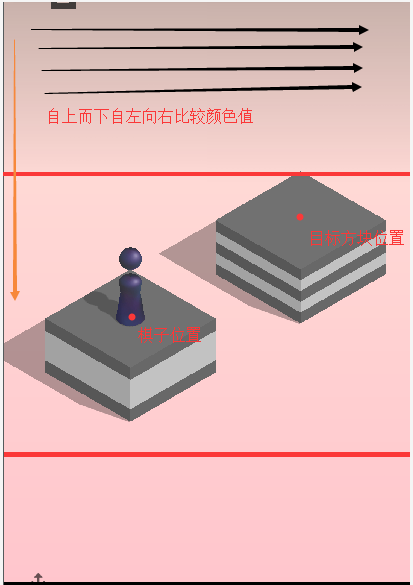# 关于微信跳一跳辅助脚本的代码学习

### 关于微信跳一跳辅助脚本的代码学习- Tools 实际上是windows需要用到的一些工具 同时打包成exe的时候也会把这块给导入
- common 一些通用的方法 这里主要是一个截屏的调用所在
- config 配置文件，主要放置不同的手机分辨率 所需要按压的参数不同
- wechat_jump_auto.py 就是android设备运行的脚本了

def main():
"""
主函数
"""
op = yes_or_no('请确保手机打开了 ADB 并连接了电脑，'
'然后打开跳一跳并【开始游戏】后再用本程序，确定开始？')
if not op:
print('bye')
return
print('程序版本号：{}'.format(VERSION))
debug.dump_device_info()
screenshot.check_screenshot()

i, next_rest, next_rest_time = (0, random.randrange(3, 10),
random.randrange(5, 10))
while True:
screenshot.pull_screenshot()
im = Image.open('./autojump.png')
# 获取棋子和 board 的位置
piece_x, piece_y, board_x, board_y = find_piece_and_board(im)
ts = int(time.time())
print(ts, piece_x, piece_y, board_x, board_y)
set_button_position(im)
jump(math.sqrt((board_x - piece_x) ** 2 + (board_y - piece_y) ** 2))
if DEBUG_SWITCH:
debug.save_debug_screenshot(ts, im, piece_x,
piece_y, board_x, board_y)
debug.backup_screenshot(ts)
im.close()
i += 1
if i == next_rest:
print('已经连续打了 {} 下，休息 {}s'.format(i, next_rest_time))
for j in range(next_rest_time):
sys.stdout.write('\r程序将在 {}s 后继续'.format(next_rest_time - j))
sys.stdout.flush()
time.sleep(1)
print('\n继续')
i, next_rest, next_rest_time = (0, random.randrange(30, 100),
random.randrange(10, 60))
# 为了保证截图的时候应落稳了，多延迟一会儿，随机值防 ban
time.sleep(random.uniform(0.9, 1.2))

def dump_device_info():
"""
显示设备信息
"""
size_str = os.popen('adb shell wm size').read()
device_str = os.popen('adb shell getprop ro.product.device').read()
phone_os_str = os.popen('adb shell getprop ro.build.version.release').read()
density_str = os.popen('adb shell wm density').read()def find_piece_and_board(im):
"""
寻找关键坐标
"""
w, h = im.size

piece_x_sum = 0  # 棋子横坐标采样点的总和
piece_x_c = 0    # 棋子采样点的总个数
piece_y_max = 0  # 棋子纵坐标采样点的最大值
board_x = 0
board_y = 0
scan_x_border = int(w / 8)  # 扫描棋子时的左右边界
scan_start_y = 0  # 扫描的起始 y 坐标
im_pixel = im.load()
# 以 50px 步长，尝试探测 scan_start_y
for i in range(int(h / 3), int(h*2 / 3), 50):
last_pixel = im_pixel[0, i]
for j in range(1, w):
pixel = im_pixel[j, i]
# 不是纯色的线，则记录 scan_start_y 的值，准备跳出循环
if pixel != last_pixel:
scan_start_y = i - 50
break
if scan_start_y:
break
print('scan_start_y: {}'.format(scan_start_y))

# 从 scan_start_y 开始往下扫描，棋子应位于屏幕上半部分，这里暂定不超过 2/3
for i in range(scan_start_y, int(h * 2 / 3)):
# 横坐标方面也减少了一部分扫描开销
for j in range(scan_x_border, w - scan_x_border):
pixel = im_pixel[j, i]
# 根据棋子的最低行的颜色判断，找最后一行那些点的平均值，这个颜
# 色这样应该 OK，暂时不提出来
if (50 < pixel < 60) \
and (53 < pixel < 63) \
and (95 < pixel < 110):
piece_x_sum += j
piece_x_c += 1
piece_y_max = max(i, piece_y_max)

if not all((piece_x_sum, piece_x_c)):
return 0, 0, 0, 0
#  对棋子的坐标做个平均取值
piece_x = int(piece_x_sum / piece_x_c)
piece_y = piece_y_max - piece_base_height_1_2  # 上移棋子底盘高度的一半

# 限制棋盘扫描的横坐标，避免音符 bug
if piece_x < w/2:
board_x_start = piece_x
board_x_end = w
else:
board_x_start = 0
board_x_end = piece_x

for i in range(int(h / 3), int(h * 2 / 3)):
last_pixel = im_pixel[0, i]
if board_x or board_y:
break
board_x_sum = 0
board_x_c = 0

for j in range(int(board_x_start), int(board_x_end)):
pixel = im_pixel[j, i]
# 修掉脑袋比下一个小格子还高的情况的 bug
if abs(j - piece_x) < piece_body_width:
continue

# 修掉圆顶的时候一条线导致的小 bug，这个颜色判断应该 OK，暂时不提出来
if abs(pixel - last_pixel) \
+ abs(pixel - last_pixel) \
+ abs(pixel - last_pixel) > 10:
board_x_sum += j
board_x_c += 1
if board_x_sum:
board_x = board_x_sum / board_x_c
last_pixel = im_pixel[board_x, i]

# 从上顶点往下 +274 的位置开始向上找颜色与上顶点一样的点，为下顶点
# 该方法对所有纯色平面和部分非纯色平面有效，对高尔夫草坪面、木纹桌面、
# 药瓶和非菱形的碟机（好像是）会判断错误
for k in range(i+274, i, -1):  # 274 取开局时最大的方块的上下顶点距离
pixel = im_pixel[board_x, k]
if abs(pixel - last_pixel) \
+ abs(pixel - last_pixel) \
+ abs(pixel - last_pixel) < 10:
break
board_y = int((i+k) / 2)

# 如果上一跳命中中间，则下个目标中心会出现 r245 g245 b245 的点，利用这个
# 属性弥补上一段代码可能存在的判断错误
# 若上一跳由于某种原因没有跳到正中间，而下一跳恰好有无法正确识别花纹，则有
# 可能游戏失败，由于花纹面积通常比较大，失败概率较低
for j in range(i, i+200):
pixel = im_pixel[board_x, j]
if abs(pixel - 245) + abs(pixel - 245) + abs(pixel - 245) == 0:
board_y = j + 10
break

if not all((board_x, board_y)):
return 0, 0, 0, 0
return piece_x, piece_y, board_x, board_y

def jump(distance):
"""
跳跃一定的距离
"""
press_time = distance * press_coefficient
press_time = max(press_time, 200)   # 设置 200ms 是最小的按压时间
press_time = int(press_time)
cmd = 'adb shell input swipe {x1} {y1} {x2} {y2} {duration}'.format(
x1=swipe_x1,
y1=swipe_y1,
x2=swipe_x2,
y2=swipe_y2,
duration=press_time
)
print(cmd)
os.system(cmd)
return press_time

# 长按的时间系数，请自己根据实际情况调节
press_coefficient = config['press_coefficient']